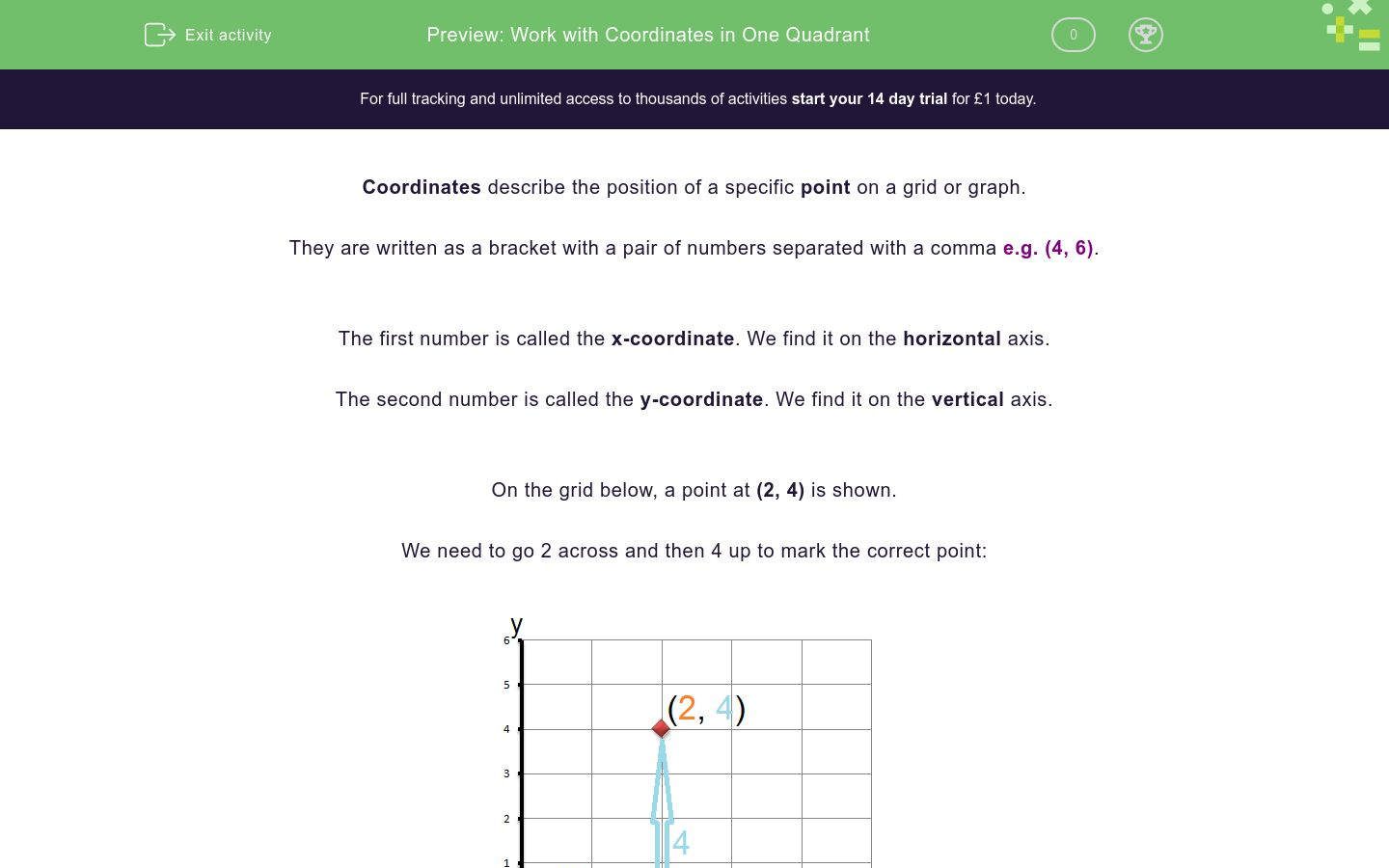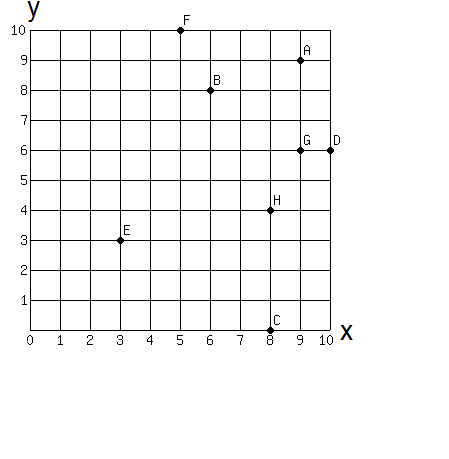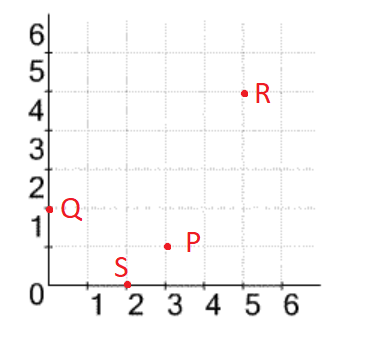# Work with Coordinates in One Quadrant

In this worksheet, students will identify and work with the coordinates of points involving positive values for x and y within one graphical quadrant.Key stage:  KS 4

GCSE Subjects:   Maths

GCSE Boards:   Pearson Edexcel, OCR, Eduqas, AQA

Curriculum topic:   Algebra, Graphs of Equations and Functions

Curriculum subtopic:   Graphs, Graphs of Equations and Functions

Difficulty level:### QUESTION 1 of 10

Coordinates describe the position of a specific point on a grid or graph.

They are written as a bracket with a pair of numbers separated with a comma e.g. (4, 6).

The first number is called the x-coordinate. We find it on the horizontal axis.

The second number is called the y-coordinate. We find it on the vertical axis.

On the grid below, a point at (2, 4) is shown.

We need to go 2 across and then 4 up to mark the correct point:A special point on the coordinate grid is the origin.

This is the point with coordinates (0, 0).

The x- and the y-axis cross at the origin.

In this activity, we will identify and work with the coordinates of points involving positive values for x and y.

You may want to have a sheet of squared paper handy, so that you can draw and map out coordinates on your own grid to help you with this activity.

Which point on the grid below has the coordinates (6, 8)?A

G

D

B

What are the coordinates of point G on the grid below?(6, 9)

(6, 6)

(9, 6)

(9, 9)

Which of the statements below are true about the point (4, 7)?

This point has an x-coordinate of 4 and a y-coordinate of 7

This point has an x-coordinate of 7 and a y-coordinate of 4

This point has x-coordinates 4 and 7

This point has y-coordinates 4 and 7

This point has a y-coordinate of 4

Consider the shape presented on the grid below:This shape can be made into a rhombus.

What are the coordinates of the fourth corner of this shape so that it becomes a rhombus?

(8 , 9)

(10, 8)

(8, 10)

(8, 11)

Match each of the points marked on the grid below with its correct coordinates.## Column B

P
(0, 2)
Q
(5, 5)
R
(3, 1)
S
(2, 0)

Which of the points listed below are not marked in red on this grid?(6, 1)

(3, 2)

(5, 6)

(1, 5)

(4, 0)

Consider the shape presented on the grid below:A third point can be added to create an isosceles triangle

What are the possible coordinates of the third corner of this shape so that it becomes an isosceles triangle?

(9, 7)

(1, 6)

(7, 6)

(7, 4)

(0, 7)

Consider the grid below:Some of the points below have an x-coordinate which is smaller than their y-coordinate (x < y), whereas others have an x-coordinate which is greater than their y-coordinate (x > y).

e.g. Point F has an x- coordinate of 2 and a y-coordinate of 7, so x < y.

Select which category each of the other points belongs to by checking the relevant box in the table below.

 x < y x > y A B C D E

True or false?

The point (3, 5) lies exactly on the x-axis.

True

False

True or false?

The coordinates of the origin are (1, 0).

True

False

• Question 1

Which point on the grid below has the coordinates (6, 8)?B
EDDIE SAYS
Let's start at 0 in the bottom, left corner. Go 6 squares to the right and then 8 squares up. This is the point with coordinates (6, 8). Which letter is attached to this point?
• Question 2

What are the coordinates of point G on the grid below?(9, 6)
EDDIE SAYS
Did you remember to count across first and then up? The correct coordinates are (9, 6). Check it yourself! We need to travel 9 squares to the right, then 6 squares up to reach point G. Practise this skill by working out the coordinates for some other points on this grid before moving on.
• Question 3

Which of the statements below are true about the point (4, 7)?

This point has an x-coordinate of 4 and a y-coordinate of 7
EDDIE SAYS
When we are working with coordinates, the first number is always the x-coordinate and the second is always the y-coordinate. So option a is the only correct statement, which accurately describes the value of each number present in the coordinate.
• Question 4

Consider the shape presented on the grid below:This shape can be made into a rhombus.

What are the coordinates of the fourth corner of this shape so that it becomes a rhombus?

(8, 10)
EDDIE SAYS
Did you remember that a rhombus is a four-sided shape with equal sides? In order to create this shape on the grid, we need to draw a corner which mirrors the lowest point so that our shape looks like a diamond or kite. This point needs to be exactly the same distance from the left and right corners on the line y = 8 as the lower point. The final corner must be at (8, 10), so 8 squares along on the x axis and 10 squares up on the y axis. Can you imagine this corner on the grid above? What will our final shape look like?
• Question 5

Match each of the points marked on the grid below with its correct coordinates.## Column B

P
(3, 1)
Q
(0, 2)
R
(5, 5)
S
(2, 0)
EDDIE SAYS
Coordinates show the position of a point. The first number in the brackets is the x-coordinate, you find it by going across the bottom axis. The second number is the y-coordinate and it is found on the vertical axis. Look at each of these points in turn and consider how many squares along and then up we need to travel to reach them. P is 3 squares along and 1 square up. Q is 0 squares along and 2 squares up. R is 5 squares along and 5 squares up. S is 2 squares along and 0 squares up. Were those easy to match?
• Question 6

Which of the points listed below are not marked in red on this grid?(3, 2)
(5, 6)
(4, 0)
EDDIE SAYS
Here you can choose to either find the coordinates of the points shown, then check them against the options in the list; OR Check each of the options in the list against the points shown on the grid. Either way, you should find that: The coordinates (3, 2), (5, 6) and (4, 0) on missing from the grid; But the points with coordinates (2, 3), (6, 5) and (0, 4) are present on the grid.
• Question 7

Consider the shape presented on the grid below:A third point can be added to create an isosceles triangle

What are the possible coordinates of the third corner of this shape so that it becomes an isosceles triangle?

(9, 7)
(0, 7)
EDDIE SAYS
Isosceles triangles are triangles with two sides of equal length. The new point needs to be halfway between the two points on the line, so along the line y = 7. Here we have a choice of creating a triangle on the left of the line already present, or the right. Therefore, any coordinates in the list with a value of 7 for the y-coordinate are valid. Remember that the y-coordinate is the second in the bracket, so (9, 7) and (0, 7) are both viable answers. Can you think of any other coordinates which would give another viable answer to this question?
• Question 8

Consider the grid below:Some of the points below have an x-coordinate which is smaller than their y-coordinate (x < y), whereas others have an x-coordinate which is greater than their y-coordinate (x > y).

e.g. Point F has an x- coordinate of 2 and a y-coordinate of 7, so x < y.

Select which category each of the other points belongs to by checking the relevant box in the table below.

 x < y x > y A B C D E
EDDIE SAYS
The coordinates of each of the points on the grid are: A = (1, 2) B = (8, 1) C = (10, 0) D = (9, 6) E = (5, 8) The first number tells us the x-coordinate, and the second tells us the y-coordinate. If the first number is smaller than the second (A and E), we can say that y is greater than x or x is less than y (x < y). If the first number is larger than the second (B, C and D), we can say that y is less than than x or x is greater than y (x > y). Did you tick those options correctly?
• Question 9

True or false?

The point (3, 5) lies exactly on the x-axis.

False
EDDIE SAYS
For a point to lie exactly on the x-axis, the y-coordinate needs to be equal to 0. Here the value of the y-coordinate (the second number) is 5, so this statement cannot be correct. It is useful to remember that a 0 in either the x- or y- coordinate value, will mean that the point will lie exactly on an axis line. Great work completing this activity! Why not level up and try a more challenging coordinates activity, if you are feeling ready for it?
• Question 10

True or false?

The coordinates of the origin are (1, 0).

False
EDDIE SAYS
The coordinates of the origin point are always (0, 0). This is an important fact to remember when working with coordinates and for the rest of this activity.
---- OR ----

Sign up for a £1 trial so you can track and measure your child's progress on this activity.

### What is EdPlace?

We're your National Curriculum aligned online education content provider helping each child succeed in English, maths and science from year 1 to GCSE. With an EdPlace account you’ll be able to track and measure progress, helping each child achieve their best. We build confidence and attainment by personalising each child’s learning at a level that suits them.

Get started